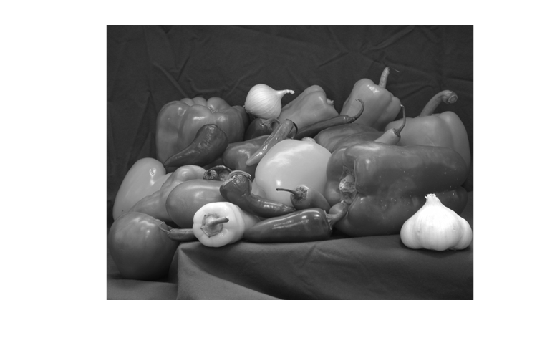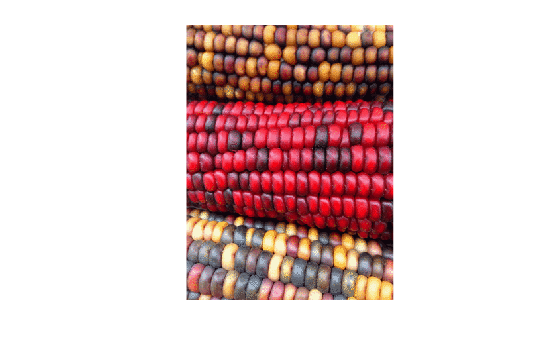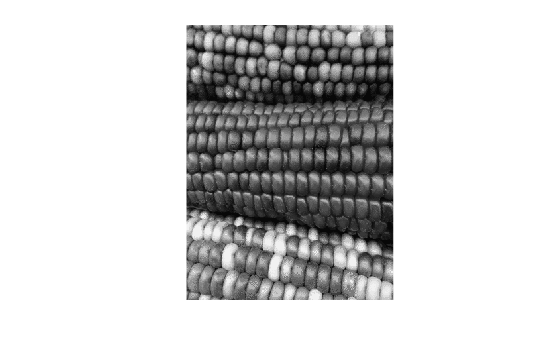# rgb2gray

Convert RGB image or colormap to grayscale

## Syntax

``I = rgb2gray(RGB)``
``newmap = rgb2gray(map)``

## Description

example

````I = rgb2gray(RGB)` converts the truecolor image `RGB` to the grayscale image `I`. The `rgb2gray` function converts RGB images to grayscale by eliminating the hue and saturation information while retaining the luminance. If you have Parallel Computing Toolbox™ installed, `rgb2gray` can perform this conversion on a GPU.```

example

````newmap = rgb2gray(map)` returns a grayscale colormap equivalent to `map`.```

## Examples

collapse all

Read and display an RGB image, and then convert it to grayscale.

Read the sample file, `peppers.png`, and display the RGB image.

```RGB = imread('peppers.png'); imshow(RGB)```Convert the RGB image to a grayscale image and display it.

```I = rgb2gray(RGB); figure imshow(I)```Read an indexed image with an RGB colormap. Then, convert the colormap to grayscale.

Read the sample file, `corn.tif`, which is an indexed image with an RGB colormap.

`[X,map] = imread('corn.tif');`

Display the image.

`imshow(X,map)`Convert the RGB colormap to a grayscale colormap and redisplay the image.

```newmap = rgb2gray(map); imshow(X,newmap)```## Input Arguments

collapse all

Truecolor image, specified as an m-by-n-by-3 numeric array.

If you have Parallel Computing Toolbox installed, `RGB` can also be a gpuArray.

Data Types: `single` | `double` | `uint8` | `uint16`

Colormap, specified as a c-by-3 numeric matrix with values in the range [0, 1]. Each row of `map` is a three-element RGB triplet that specifies the red, green, and blue components of a single color of the colormap.

If you have Parallel Computing Toolbox installed, `map` can also be a gpuArray.

Data Types: `double`

## Output Arguments

collapse all

Grayscale image, returned as an m-by-n numeric array.

If you have Parallel Computing Toolbox installed, then `I` can also be a gpuArray.

Grayscale colormap, returned as an c-by-3 numeric matrix with values in the range [0, 1]. The three columns of `newmap` are identical, so that each row of `map` specifies a single intensity value.

If you have Parallel Computing Toolbox installed, then `newmap` can also be a gpuArray.

Data Types: `double`

## Tips

• The `rgb2gray` function returns an error if the input image is a grayscale image. The `im2gray` function is identical to `rgb2gray` except that it can accept grayscale images as inputs, returning them unmodified. If you use the `im2gray` function, code like this conditional statement is no longer necessary.

```if ndims(I) == 3 I = rgb2gray(I); end```

## Algorithms

`rgb2gray` converts RGB values to grayscale values by forming a weighted sum of the R, G, and B components:

```0.2989 * R + 0.5870 * G + 0.1140 * B ```

These are the same weights used by the `rgb2ntsc` (Image Processing Toolbox) function to compute the Y component.

The coefficients used to calculate grayscale values in `rgb2gray` are identical to those used to calculate luminance (E'y) in Rec.ITU-R BT.601-7 after rounding to 3 decimal places.

Rec.ITU-R BT.601-7 calculates E'y using the following formula:

```0.299 * R + 0.587 * G + 0.114 * B ```